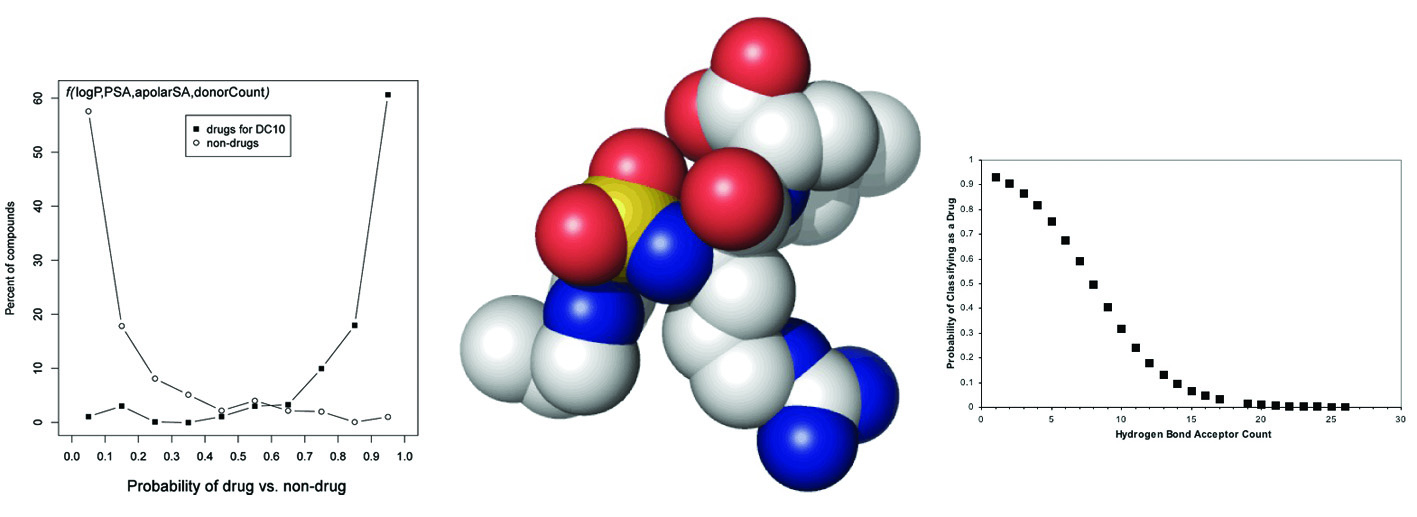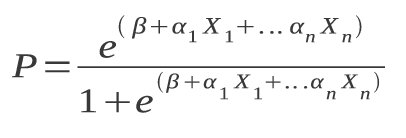### Probability of Drugs and Non-Drugs

Predicting the probability of a compound being classified as a drug or non-drug, as well as disease category (or organ) classification (DC).### Functions for calculating drug, non-drug and DC classification.

The following logistic regression functions are based on the formula:and are described in A.T. Garcia-Sosa,* M. Oja, C. Hetenyi, U. Maran, "DrugLogit: Logistic Discrimination between Drugs and Nondrugs Including Disease-Specificity by Assigning Probabilities Based on Molecular Properties", Journal of Chemical Information and Modeling, 2012, Vol. 52, Iss. 8, 2165-2180. DOI: http://dx.doi.org/10.1021/ci200587h , [PDF].
The data used to obtain the functions is available in the Supporting Information to this paper, available here.
If you use the calculators or functions, or modify them, please cite this paper.

You can use the DrugLogit calculators© below to type in the values of the properties of compounds and compute their drug probability value according to any of the equations 3 through 10 of the article and this page. The non-drug probability is 1 − Pdrug.

The DC probability is computed using equations 14 through 23.

The equations can be chosen based on their individual prediction accuracy, or the relevance and availability of their physicochemical property variables to a given use case. They can also be used to filter compounds by applying one or several of the equations in hierarchical (i.e., successively applied) filters, or by chosing compounds that are predicted in concensus by two or more equations. It is important to note that due to the small number of variables in the equations, they remain immediately interpretable in chemical and physical terms.

Molecular properties of compounds can be easily calculated using, for example, Marvin, or looked-up in databases such as PubChem, or others. The equations were derived using Marvin and calculator plugins from ChemAxon, and logP values calculated by the atom-additive method in XLogP.

Abbrev.
Acceptors and Donors are the number of hydrogen bond acceptors and donors, resp., in the molecule.
Bonds is the total number of bonds in the molecule.
PSA, apolarSA, and MSA are, resp., the polar, apolar, and total molecular surface area in Å2.
Rings is the total number of rings in the molecule.
logP is the logarithm (base 10) of the octanol/water partition function.
MW is the molar mass (or exact mass) of a molecule in g/mol.
NHA is the number of heavy atoms (i.e., non hydrogen atoms) in the molecule.
ΔG is the binding free energy in kcal/mol.

Note: A value of NaN indicates a very small value, close to zero.

Functions to calculate the probability of classifying as a drug vs. non-drugs.

#### Eq. 3

 Acceptors

Pdrug value:

#### Eq. 4

 PSA

Pdrug value:

#### Eq. 5

 Rings PSA

Pdrug value:

#### Eq. 6

 Aliphatic rings logP MW

Pdrug value:

#### Eq. 7

 Aliphatic rings PSA Bonds

Pdrug value:

#### Eq. 8

 logP Wiener index PSA

Pdrug value:

#### Eq. 9

 Balaban index Aromatic rings Donors ΔG NHA

Pdrug value:

#### Eq. 10

 Aliphatic rings logP PSA ΔG Wiener index

Pdrug value:

___________________

Functions to calculate the probability of classifying as a specific DC drug vs. drugs from other DCs.

#### Eq. 14

Probability of drug belonging to DC8
(Anti-Neoplastic agents) as opposed to other DCs.

 Number of Carbons PSA Molecular polarizability

PdrugDC8 value:

#### Eq. 15

Probability of drug belonging to DC10
(Nervous system) as opposed to other DCs.

 Aromatic rings Aliphatic rings Number of Carbons PSA ΔG MSA

PdrugDC10 value:

___________________

Functions to calculate the probability of classifying as a specific DC drug vs. non-drugs.

#### Eq. 16

Probability of drug belonging to DC1
(Alimentary system and Immonomodulating)
vs. non-drugs.

 Aromatic rings PSA ΔG ApolarSA

PdrugDC1 value:

#### Eq. 17

Probability of drug belonging to DC3
(Cardiovascular system) vs. non-drugs.

 Balaban index logP MSA Aromatic atoms

PdrugDC3 value:

#### Eq. 18

Probability of drug belonging to DC5
(Genito-Urinary system) vs. non-drugs.

 Aromatic rings PSA Wiener polarity

PdrugDC5 value:

#### Eq. 19

Probability of drug belonging to DC7
(Anti-Infectives) vs. non-drugs.

 Rings Number of Carbons PSA Wiener polarity

PdrugDC7 value:

#### Eq. 20

Probability of drug belonging to DC10
(Nervous system) vs. non-drugs.

 logP PSA ApolarSA Donors

PdrugDC10 value:

#### Eq. 21

Probability of drug belonging to DC12
(Respiratory system) vs. non-drugs.

 Aromatic rings hyper Wiener index ΔG ApolarSA

PdrugDC12 value:

#### Eq. 22

Probability of drug belonging to DC13
(Sensory system) vs. non-drugs.

 logP PSA ΔG Number of Carbons

PdrugDC13 value:

#### Eq. 23

Probability of drug belonging to DC14
(Various drugs) vs. non-drugs.

 Donors ΔG ApolarSA

PdrugDC14 value:

___________________

Garcia-Sosa A.T.,* Oja M., Hetenyi C., Maran U., "DrugLogit: Logistic Discrimination between Drugs and Non-drugs Including Disease-Specificity by Assigning Probabilities Based on Molecular Properties", Journal of Chemical Information and Modeling, 2012, Vol. 52, Iss. 8, 2165-2180. DOI: http://dx.doi.org/10.1021/ci200587h , [PDF].

See Publications.